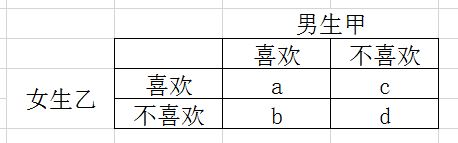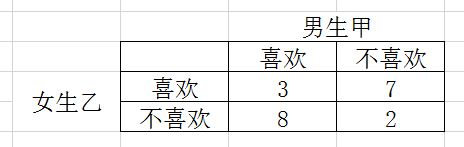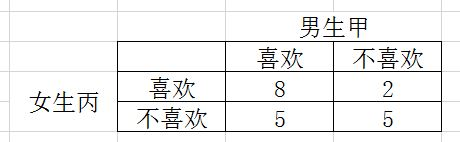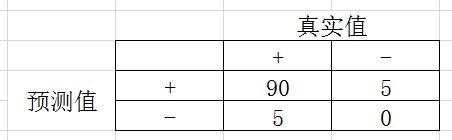kappa statistic怎么理解？有什么意义？

统计/机器学习 模型验证 描述性统计    浏览次数：8408        分享

20

kappa statistic怎么理解？有什么意义？想当小五哥   2017-03-11 12:26

2个回答
38

Kappa值或者Kappa系数是用来度量两个被观测对象的一致程度。一般用希腊字母$\kappa$来表示。

$\kappa$是如何计算的？我们直接上栗子。假设我们有两个对象，对，对象，男生甲和女生乙，相亲。媒婆想知道他们两个能不能处得来，首先就想问能不能吃到一块去。就分别问两个人二十道菜，他们只需回答“喜欢”或者“不喜欢”这道菜。媒婆非常认真，做了下面的表，$a$是男生喜欢、女生也喜欢吃的菜的数量，$b$是男生喜欢、女生不喜欢的菜的数量，以此类推。

Kappa值的计算公式如下

$$\kappa=\frac{\frac{a+d}{a+b+c+d}-\frac{(a+c)\times(a+b)+(b+d)\times(c+d)}{(a+b+c+d)^2}}{1-\frac{(a+c)\times(a+b)+(b+d)\times(c+d)}{(a+b+c+d)^2}}$$

$\kappa$的值在$-1$$1之间。 越接近1，两者越一致、越吻合。换句话说，男生甲和女生乙是有缘人！我们喜欢一样的东西，也讨厌一样的东西。 接近0，表面两者之间符合偶然的预期。换句话说，男生甲和女生乙是路人！ 接近-1，表面两者之间的相符程度非常低。换句话说，男生甲和女生乙是冤家，死对头！我喜欢你讨厌的东西，我讨厌你喜欢的东西。 具体来算两个例子。$$\kappa=\frac{\frac{3+2}{20}-\frac{11\times 10+10\times 7}{20^2}}{1-\frac{11\times 10+10\times 7}{20^2}}=-0.5$\$SofaSofa数据科学社区DS面试题库 DS面经高代兄   2017-03-17 08:09

15

kappa又叫做Cohen's kappa。可以用来衡量一个二元分类器的准确性，而且非常实用于标签非平衡的情况。

kappa的数值在-1到1之间。越大说明分类器越好。

kappa = 0，说明这个分类器和随便猜测没什么区别。这里的猜测是按照整体的分布进行猜测。比如已知90%的标签是1，你也是按照这个概率随机猜测。如果按照其他概率来猜测，得到的kappa很可能是负数。

kappa > 0.1，说明模型凑合，马马虎虎

kappa > 0.4，说明模型还行

kappa > 0.8，说明模型挺好的

------补充------

SofaSofa数据科学社区DS面试题库 DS面经sasa   2017-09-21 05:47相关主题